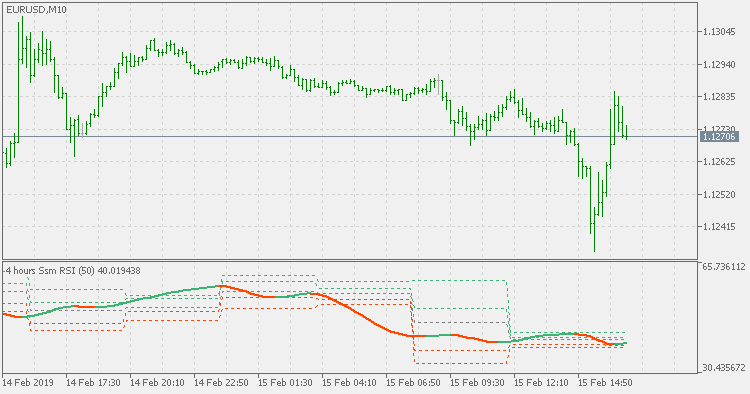Interesting script?
So post a link to it -
let others appraise it

You liked the script? Try it in the MetaTrader 5 terminal# SSM RSI pivots - indicator for MetaTrader 5

Views:
1252
Rating:
Published:
2019.02.15 16:06

Theory :

The original calculation of RSI is (in short) the following :

Add the changes up and changes  down. From those sums, we can then calculate the RSI (Relative Strength Index). At that particular step Welles Wilder used what sometimes is called Wilders EM for smoother results, And that gives us space to [produce all sort of different RSIs by using different types of smoothing at the final step

This version :

It is using super smoother for RSI calculation. It produces results similar to RSX (that was invented by Mark Jurik) but the differences are significant enough to have it as a separate type of RSI

Also, this version is adding pivot calculation to such RSI. Pivots are calculated from the RSI itself, and you have 4 possible types that can be displayed :

• display pivot only
• display pivot and 1st level support and resistance
• display pivot and 1st and 2nd level support and resistance
• display pivot and 1st and 2nd and 3rd level support and resistance

The time frame selected, is used for pivots only (that time frame is used as "delimiter" for pivots). The RSI is always calculated as current time frame

Usage :

You can use it as any other RSISimple support resistance

Simple support resistanceSimple intraday support resistance

Simple intraday support resistanceRocket RSI pivots

Rocket RSI pivotsTrix pivots

Trix pivots